L'état stable

# Information and Stability

(for French, click on the flag)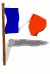## 1/ Definition of a stable state:

As per definition, the stable state of a System is these one the system reaches when it evolves naturally, by itself.
Boltzman's approach lays on defining the stable state of a system as the one which can be reach by the greatest different possible ways.
This definition is quite simple, it comes from the following idea : if you take a way at random, your luck to arrive on a particular place is a direct function of the number of ways arriving there.

Let us consider a very simple system, constituted by a number of elements spread in a collection of boxes.
We suppose the elements identical, but without any interaction between them.
The simplest example is a system constituted by two boxes, on which n elements are disposed (of course n>2).
Following Planck, we call "complexion", a particular distribution of the elements within the boxes.

One complexion remains unchanged if we permutate two elements : we just take into account the number of elements within the boxes.

When we say the elements are "identical", that means their similarity is sufficient enough for our purpose.
We will now calculate the probability to obtain any complexion.

## 2/ Number of Complexions:

As said previously, we cannot distinguish the elements between them, so a permutation between two elements give the same complexion.

Let us use "0" as an image of one element, and represent the separation between our two boxes by a "/".
For 5 elements spread between 2 boxes the possibilities are as follows :
00000/
0000/0
000/00
00/000
0/0000
/00000
In the given example, there is six possible complexions.
Generally speaking, to arrange n elements within s boxes we can, as done previously, align them and put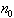in the first box,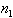in the second one and so on.
Doing so, we obtain a particular complexion.
As we cannot distinguish two elements between them, there is as many ways to obtain this specific complexion as many possibilities to permutate the n elements between them to form the initial alignment.
The number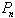of possibilities to permutate n elements is easily deducted from the number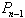of permutations of n-1 elements.
As a matter of fact, in an alignment of n-1 elements, there is n possibilities to introduce a new element : it could be between two of them (n-2 possibilities), or at one of the two ends (2 possibilities).
So :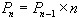Step by step, it comes: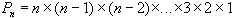Expression noted : n! (factorial n).

So, there is n! ways to arrange n elements in s boxes in a specific order.

But, for a given complexion in which the nth boxe contains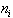element, we don't care about the order of the elements within it.
The number of permutations inside the box i is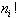As a result, for the box i,distinct arrangements give the same complexion (as per our definition).
So, the number of different ways to obtain the specific complexion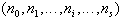of n elements within s boxes is :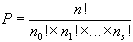In our example of a system made of 2 boxes: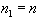;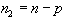(where n
is the total number of elements).
In that conditions, it is easy to see that the number of ways to realize a specific complexion is a function of p :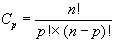## 3/ Probability to obtain any repartition:

We call "repartition mode" each different classification, either because the number of elements in the boxes is different or in the case there number are the same, because the elements itselves are not the same.
For the first element there is two possibilities: to be on the left box or to be on the right one, for the second element, there is also two possibilities. So for the two elements together, there is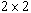possibilities.
Step by step we set up that there is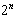possible repartition modes of n elements within two boxes.
So, for any repartition mode, there is 1 possibility uponpossible cases.
If we have no specific information we can consider that every occurrence has the same probability to be obtained.
There is only one possibility uponpossible cases :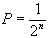## 4/ Probabilité to obtain any complexion:

Every repartition mode, giving the same particular complexion has the same probability, so, the probability to obtain a particular complexion, is in our example :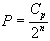Asis constant, the most probable complexion is the one for which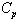is maximum,
That means that :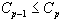and: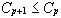For our example, with two different boxes: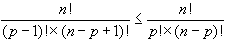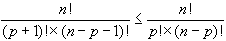After simplification :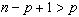et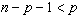If n is even :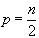; if not :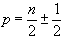That means the stable state is the one where the number of elements into the boxes is as equal as possible.
Calculus show that the probability to obtain a given complexion near the stable one decreases sharply when n increases.

## 5/ Observation stability:

Stable state of a system is the one for which the realisability (as per our previous definition) is maximum, in other words the one which can be reached (or realized) by the maximum different ways.

In fact, this definition of the stability is depending of the Observer's intention.

To be more precise, let us consider two different observers of a system composed of n elements within two boxes.
The first observer considers each of the n elements as distinct individuals, when the second one considers the elements are equivalent.
The first observer is not interested by the "complexions", but by the "repartition modes" of the elements. He is able to distinguish a single permutation between two elements.
So, for this observer, the system presents a number of potential states equals to, which is the number of different repartition modes the elements can achieve.
Moreover, all the states have the same probability which is equals to :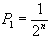.
In other words, our first observer doesn't see any specific state which can be seen as "stable".

Let us now consider the second one, who can only consider the different "complexions" of the system considered. We have seen that, for our example, their number is n+1.
Except the case where n=1, it is obvious that the first observer has more work than the second one, for describing the system (more different states to consider)
The work of our second observer is even easier due to the fact that for him, the different states of the system have not the same probability. He is able to define as a "stable state" the one where the elements are distributed equally between the two boxes.
This stable state has a probability of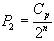to occur.

This is, from my point of view the quite "magic side" of Boatman's thought.

It shows us that, when we renounce to detail, to differentiate, when we accept our perception of details grows poorer, we improve our understanding of the whole system. At a more global scale, we extricate some stable forms, we built a representation.

However, the difference between our two observers is only due to the fact their observations have not the same accuracy.

In fact, the observer n°1 as well as the observer n°2 has no direct access to the system he observes. To say observer n°1 can, within a total of n, distinguishes one element from the other means only that he can arrange them on a grid offering more than n possibilities.
Inherently, our two observers have done the same kind of work to characterize the elements, to "classify " them, but each of them with at a different scale :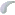First one characterizes the n elements according to at least n criteria (upon which their membership of a set of our two boxes)The second one has only to classify them regarding their distribution within our two boxes.
This passage from one analysis level to the other structured our vision of the system, gives him a "form" and creates, in the etymological sense of the word some "information".

Distinguishing a new state as the "stable state" of the system, the observer n°2 adds a new information, impossible to deduct from the description giving by the observer n°1.

N°2 Observer's discourse is a "meta-discourse" in comparison with N°1 Observer's one.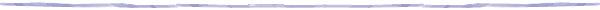page updated on 02/02/99
author : Alain SIMONnote 1:
This leads us back to Michel Foucault in "Les Mots et les Choses".
He shows in fact how the first concern of a science at its beginning is to "define criteria" and to "classify" the observations following these criteria.
This thought process, when it succed to point out some stable observations, is trully a "source of information".
For example, the Meneleiev classification gave such information.
Taxinomy is a major way to estabish "rules".
As a matter of fact, "our" natural laws are only the result of some stable distribution within different grids.
return